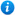Decimal Tenths on a Number Line - Year 4

Decimal Tenths on a Number Line - Year 4

Year 4 maths programme of study - Number - fractions (including decimals)

• recognise and write decimal equivalents of any number of tenths

In this KS2 maths teaching resource pupils practise reading and representing decimal tenths on a number line. It is an ideal teaching aid to use in a lesson covering the year 4 curriculum objective listed above. Content includes:

• Identifying the decimal tenth indicated on the number line activity and worksheet
• Placing the correct decimal number on to the number line activity and worksheet
• Identifying the decimal tenth indicated on the number line activity and worksheet
• Placing the correct decimal number on to the number line activity and worksheet
• Completing the number lines activity and worksheet
• Reasoning and problem solving activity and worksheet

'Decimal Tenths on a Number Line - Year 4' is editable so teachers can adapt the resource to meet their individual teaching needs.

Click on the images opposite to view 'Decimal Tenths on a Number Line - Year 4' in full.

Our Price : £1.99 / 2 CreditsGet this resource as part of a bundle and save up to 55%Year 4 Decimals Bundle

£9.99

By continuing to use the site, you agree to the use of cookies. You can change this and find out more by following this link